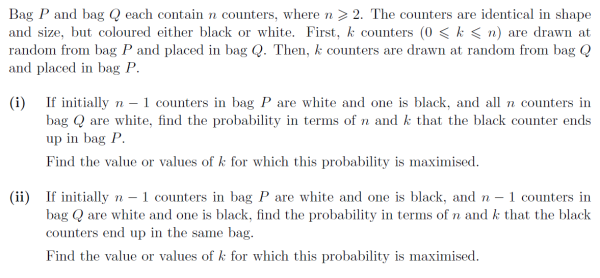# Worked Example: Counters in Bags

##### Age 16 to 18Challenge Level

Now we have reviewed the key concepts behind Probability in STEP, lets test ourselves with an example. Below is Question 13 from STEP II 2008:This question is a particularly useful one for illustrating that often, all we need to do is count.

Let's begin by determining what we need to calculate in part (i): how can the single black counter that begins in bag $P$, end in bag $P$ after the two moves. Well, either it was moved from $P$ to $Q$ and then moved back again, or it simply remained in bag $P$ and was never moved. Therefore, we actually have:

$\hspace{0.5 in} \mathbb{P}(\text{Counter ends in Bag} \hspace{0.05 in} P)=\mathbb{P}(\text{Counter Moves to Bag} \hspace{0.05 in} Q \hspace{0.05 in} \text{and back to Bag} \hspace{0.05 in} P)$
$\hspace{3.5 in} +\mathbb{P}(\text{Counter remains in Bag} \hspace{0.05 in} P).$

So, viewing this as a counting problem we now need to think about the number of ways these two scenarios can occur, out of all possible counter moves. Let's begin with the latter: how many ways can $k$ counters be chosen to move to Bag $Q$ from the $n-1$ left when we know the black counter isn't selected? Well, we dealt with this exact issue earlier. It's simply:

$\hspace{3.2 in} {}^{n-1}C_k={n-1 \choose k}.$

And how many total possible selections of $k$ counters are there from the $n$? Well that's just:

$\hspace{3.28 in} {}^{n-1}C_k={n \choose k}.$

Putting these two results together we have:

$\hspace{1.7 in} \mathbb{P}(\text{Counter remains in Bag} \hspace{0.05 in} P)=\frac{n-1 \choose k}{n \choose k}=\frac{n-k}{n},$

after some simplifying.

Now we turn out attention to the former scenario. To begin, let's observe that this probability splits up nicely as:

$\hspace{0.3 in} \mathbb{P}(\text{Counter Moves to Bag} \hspace{0.05 in} Q \hspace{0.05 in} \text{and back to Bag} \hspace{0.05 in} P)=\mathbb{P}(\text{Counter Moves to Bag} \hspace{0.05 in} Q)$
$\hspace{2.5 in} \times \mathbb{P}(\text{Counter Moves back to Bag} \hspace{0.05 in} P | \text{It moved to Bag} \hspace{0.05 in} Q).$

So what's the probability we choose $k$ counters including the single black one and move them to bag $Q$? Analagously to the probability computed above, we just need to consider how many ways there are to choose $k-1$ counters out of $n-1$ (the $k^\text{th}$ one moved is the black one), and compare this to the total number of ways to choose $k$ counters from $n$:

$\hspace{2.1 in} \mathbb{P}(\text{Counter moves to Bag} \hspace{0.05 in} Q)=\frac{n-1 \choose k-1}{n \choose k}.$

Finally, what is the probability that we choose $k$ counters including the black one from bag $Q$ (which now contains $n+k$ counters) and move them to bag $P$? Hopefully, you should be able to see from the above that that's just:

$\hspace{1 in} \mathbb{P}(\text{Counter moves back to Bag} \hspace{0.05 in} P|\text{It moved to Bag} \hspace{0.05 in} Q)=\frac{n+k-1 \choose k-1}{n+k \choose k}.$

Thus the probability of the first scenario of interest is:

$\hspace{0.7 in}\mathbb{P}(\text{Counter Moves to Bag} \hspace{0.05 in} Q \hspace{0.05 in} \text{and back to Bag} \hspace{0.05 in} P)=\frac{n-1 \choose k-1}{n \choose k} \frac{n+k-1 \choose k-1}{n+k \choose k}=\frac{k^2}{n(n+k)}.$

Thus, we can finally compute our original required probability! We have:

$\hspace{1.7 in} \mathbb{P}(\text{Counter ends in Bag} \hspace{0.05 in} P)=\frac{k^2}{n(n+k)}+\frac{n-k}{n}=\frac{n}{n+k}.$

Before we move on to part (ii) we're tasked with determining for what value of $k$ this is maximised. It should be fairly clear that this is decreasing in $k$, thus we want $k=0$. Alternatively, we can view this as a function of $k$ and differentiate to find the maxima:

$\hspace{1.5 in} f(k)=\frac{n}{n+k} \Rightarrow f'(k)=-\frac{n}{(n+k)^2}<0 \hspace{0.05 in} \text{for all} \hspace{0.05 in} 0 \leq k \leq n,$

and so again we find $k=0$ will maximise our expression.

So, now on to part (ii). Here we have two black counters: one in each Bag, and need to compute the probability they end up in the same Bag. Proceeding as in part (i), we consider how this can happen. Now we have three scenarios contributing to this probability:
• Scenario 1: The black counter in Bag $P$ was moved to $Q$ and then both moved back to $P$,
• Scenario 2: The black counter in Bag $P$ was moved to $Q$ and then both remain in $Q$,
• Scenario 3: The black counter in Bag $P$ is not moved and the black counter from Bag $Q$ is moved to $P$.
Computing the probability for each of these scenarios is then done by counting exactly like in part (i). You should be able to convince yourself that we have:

\eqalign{ \hspace{1 in} \mathbb{P}(\text{Scenario} \hspace{0.05 in} 1) &= \frac{n-1 \choose k-1}{n \choose k} \frac{n+k-2 \choose k-2}{n+k \choose k}=\frac{k^2(k-1)}{n(n+k)(n+k-1)}, \cr \mathbb{P}(\text{Scenario} \hspace{0.05 in} 2) &= \frac{n-1 \choose k-1}{n \choose k} \frac{n+k-2 \choose k}{n+k \choose k}=\frac{k(n-1)}{(n+k)(n+k-1)}, \cr \mathbb{P}(\text{Scenario} \hspace{0.05 in} 3) &= \frac{n-1 \choose k}{n \choose k} \frac{n+k-1 \choose k-1}{n+k \choose k}=\frac{k(n-k)}{n(n+k)}. }

Thus, our required probability is:

$\hspace{0.8 in} \mathbb{P}(\text{Both Counters End in Same Bag})=\frac{k^2(k-1)}{n(n+k)(n+k-1)} + \frac{k(n-1)}{(n+k)(n+k-1)}$
$\hspace{4.7 in} + \frac{k(n-k)}{n(n+k)},$
$\hspace{3.92 in} =\frac{2k(n-1)}{(n+k)(n+k-1)}.$

To finish, we again need to compute the value of $k$ maximises this probability. Here, we turn to differentiation:

$\hspace{2 in} g(k)=\frac{2k(n-1)}{(n+k)(n+k-1)} \Rightarrow g'(k)=\frac{n^2-n-k^2}{(n+k)^2 (n+k-1)^2}.$

Since the numerator above is a negative quadratic in k, our maxima of interest will occur at the positive root, i.e. at $\sqrt{n(n-1)}$. But, this isn't an integer; and $k$ must be. Thus, our actual $k$ to maximise the probability will be one of the integers either side of this root.

And that's the question done! The counting involved was indeed complicated, but as noted earlier, it was only that: counting. With practice you can quickly become more adept at computing such probabilities yourself, and once you are, probability questions can definitely be your friend in STEP.